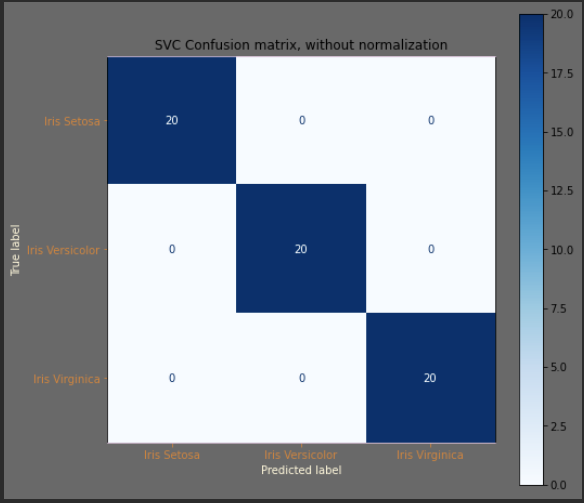top of page
Search
• Tim Burns

# Exploring Classifiers in Machine Learning

Updated: Jul 5, 2021Today, my ML path has me learning Decision Tree Classifiers. All the visualizations I did yesterday focused on looking at flower data and visualizing how the data mapped to Iris species.

I use a handy-dandy set_colors function to label all my work with Darkula colors in PyCharm.

```#%%
def set_colors(current_axis, x_axis="X-axis", y_axis="Y-axis"):
"""
The set_colors is a standard setting I use to give nice colors when
running Jupyter in Darkula mode on PyCharm.

:param current_axis:
:param x_axis:
:param y_axis:
:return:
"""
current_axis.set_xlabel(x_axis)
current_axis.set_ylabel(y_axis)
current_axis.spines['bottom'].set_color('thistle')
current_axis.spines['top'].set_color('thistle')
current_axis.xaxis.label.set_color('Cornsilk')
current_axis.yaxis.label.set_color('Cornsilk')
current_axis.tick_params(axis='x', colors='peru')
current_axis.tick_params(axis='y', colors='peru')
current_axis.set_facecolor('WhiteSmoke')```

Assign the column names and plot historgraphs on the diagonal for each of the metrics, then compare metrics to see how visually, the different properties of each species separate one from another.

```#%%
graph = sns.pairplot(train, hue="label", height = 2, palette = 'colorblind');
for ax in range(0, 4):
for ay in range(0, 4):
set_colors(graph.axes[ax,ay], x_axis=column_names[ax], y_axis=column_names[ay])

```### The Decision Tree Classifier

The Decision Tree Classifier runs iteratively through the data set and divides it into boxes that minimize Entropy and maximize Information Gain at every step.

Entropy (see the previous blog article) measures the randomness of a system. Information Gain is a term for picking a set of samples in an iteration to lose the most randomness and get the most Information.

I use Pandas to run the decision tree algorithm, and it will provide an absolute measure of how each property contributes to the classification on a scale from 0 to 1.

```#%%
import matplotlib.pyplot as plt
from sklearn.tree import plot_tree

fig, axes = plt.subplots(1, 1)
fig.patch.set_facecolor('DimGray')
plot_tree(mod_dt, feature_names = column_names, class_names = label_names, filled = True);```The visualize tool provides an excellent resource for QA on the model. Finally, after four iterations on the model, the classification finds the important metrics to determine the Iris flower species.

Run the Confusion Matrix to QA the model and determine if it misclassifies any data points.

```#%%
# The confusion matrix helps to show how our model will handle new values
from sklearn.metrics import plot_confusion_matrix
fig, ax = plt.subplots(figsize=(8, 8))
fig.patch.set_facecolor('DimGray')
set_colors(ax)
ax.set_title('Decision Tree Confusion matrix, without normalization');
plot_confusion_matrix(mod_dt, X_test, y_test,
display_labels=label_names,
cmap=plt.cm.Blues,
ax=ax,
normalize=None)

plt.show()```We can do better. The workhorse for the data classification is the Support Vector Machine or SVM set of algorithms. A good video describing Support Vector algorithms is here. Essentially, it boils our previous iterative problem into maximizes the distance between the classification borders.

Applying the SVM Support Vector Classifier to the data gives a better model that doesn't misclassify the row in the previous model.

```#%%
from sklearn.svm import SVC

svc_model = SVC(gamma='auto')
svc_model.fit(X_train,y_train)
prediction=svc_model.predict(X_test)
print("The accuracy of the SVC Tree is","{:.3f}".format(metrics.accuracy_score(prediction,y_test)))

#%%
# The confusion matrix helps to show how our model will handle new values
fig, ax = plt.subplots(figsize=(8, 8))
fig.patch.set_facecolor('DimGray')
set_colors(ax)
ax.set_title('SVC Confusion matrix, without normalization');
plot_confusion_matrix(svc_model, X_test, y_test,
display_labels=label_names,
cmap=plt.cm.Blues,
ax=ax,
normalize=None)

plt.show()```Voila- all points predicted correctly.

Concluding my exploration, analyzing data with Pandas and Scikit, visualizing data helps to perform quality analysis on a data model. Many methods exist and determining the model accuracy, and finding the best model in an interactive environment leads to a foundational understanding you can use to implement ML in an industrial-scale environments like Spark or DataBricks.